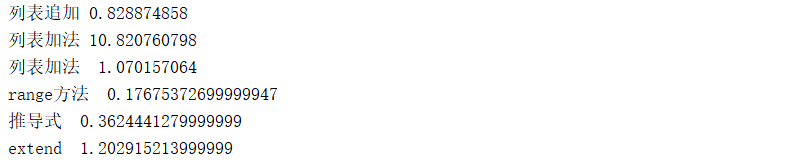• ## python列表添加数据

万次阅读 2018-11-19 16:18:28
python列表里面添加数据的方法以及各种方法时所用时间 循环 arr=[] for item in range(1000): arr.append(item) 加法 arr=[] for item in range(1000): arr=arr+[item] range的方法 list(range(1000)) ...
python往列表里面添加数据的方法以及各种方法时所用时间

循环

def aa():
arr = []
for a in range(1000):
arr.append(a)

print("列表追加 "+str(timeit.timeit('aa()',setup="from __main__ import aa",number=10000)))


加法

def bb():
arr=[]
for a in range(1000):
arr=arr+[a]

print("列表加法 "+str(timeit.timeit('bb()',setup="from __main__ import bb",number=10000)))


range的方法

def dd():
return list(range(1000))
print("range方法  "+str(timeit.timeit('dd()',setup="from __main__ import dd",number=10000)))


推导式

def ee():
return [i for i in range(1000)]
print("推导式  "+str(timeit.timeit('ee()',setup="from __main__ import ee",number=10000)))

5.extend
def ff():
arr=[]
for item in range(1000):
arr.extend(["item"])
print("extend  "+str(timeit.timeit('ff()',setup="from __main__ import ff",number=10000)))

6.加等于
def cc():
arr=[]
for a in range(1000):
arr+=[a]

print("列表加法  "+str(timeit.timeit('cc()',setup="from __main__ import cc",number=10000)))

运行结果通过比较,range方法运行效率高


展开全文运行效率
• python 数组添加数组Python doesn’t have any specific data type as an array. We can use List that has all the characteristics of an array. Python没有任何特定的数据类型作为数组。 我们可以使用具有数组...
python 数组添加数组Python doesn’t have any specific data type as an array. We can use List that has all the characteristics of an array. Python没有任何特定的数据类型作为数组。 我们可以使用具有数组所有特征的List。
Python array module can be used to create an array of integers and floating-point numbers. Python数组模块可用于创建整数和浮点数的数组。

If you want to do some mathematical operations on an array, you should use the NumPy module. 如果要对数组进行一些数学运算，则应使用NumPy模块。
1. Python添加到数组 (1. Python add to Array)
If you are using List as an array, you can use its append(), insert(), and extend() functions. You can read more about it at Python add to List. 如果将List用作数组，则可以使用其append（），insert（）和extend（）函数。 您可以在Python add to List中阅读有关它的更多信息。 If you are using array module, you can use the concatenation using the + operator, append(), insert(), and extend() functions to add elements to the array. 如果使用的是数组模块，则可以使用+运算符，append（），insert（）和extend（）函数进行串联，以将元素添加到数组中。 If you are using NumPy arrays, use the append() and insert() function. 如果您使用的是NumPy数组，请使用append（）和insert（）函数。  2.使用数组模块将元素添加到数组 (2. Adding elements to an Array using array module)
Using + operator: a new array is returned with the elements from both the arrays. 使用+运算符：返回一个新数组，其中包含两个数组中的元素。 append(): adds the element to the end of the array. append（）：将元素添加到数组的末尾。 insert(): inserts the element before the given index of the array. insert（）：将元素插入到数组的给定索引之前。 extend(): used to append the given array elements to this array. extend（）：用于将给定的数组元素附加到此数组。 import array

arr1 = array.array('i', [1, 2, 3])
arr2 = array.array('i', [4, 5, 6])

print(arr1)  # array('i', [1, 2, 3])
print(arr2)  # array('i', [4, 5, 6])

arr3 = arr1 + arr2
print(arr3)  # array('i', [1, 2, 3, 4, 5, 6])

arr1.append(4)
print(arr1)  # array('i', [1, 2, 3, 4])

arr1.insert(0, 10)
print(arr1)  # array('i', [10, 1, 2, 3, 4])

arr1.extend(arr2)
print(arr1)  # array('i', [10, 1, 2, 3, 4, 4, 5, 6]) 3.向NumPy数组添加元素 (3. Adding elements to the NumPy Array)
append(): the given values are added to the end of the array. If the axis is not provided, then the arrays are flattened before appending. append（）：将给定值添加到数组的末尾。 如果未提供轴，则在附加之前将阵列弄平。 insert(): used to insert values at the given index. We can insert elements based on the axis, otherwise, the elements will be flattened before the insert operation. insert（）：用于在给定索引处插入值。 我们可以基于轴插入元素，否则，将在插入操作之前将元素展平。 >>> import numpy as np
>>> np_arr1 = np.array([[1, 2], [3, 4]])
>>> np_arr2 = np.array([[10, 20], [30, 40]])
>>>
>>> np.append(np_arr1, np_arr2)
array([ 1,  2,  3,  4, 10, 20, 30, 40])
>>>
>>> np.append(np_arr1, np_arr2, axis=0)
array([[ 1,  2],
[ 3,  4],
[10, 20],
[30, 40]])
>>>
>>> np.append(np_arr1, np_arr2, axis=1)
array([[ 1,  2, 10, 20],
[ 3,  4, 30, 40]])
>>>
>>> np.insert(np_arr1, 1, np_arr2, axis=0)
array([[ 1,  2],
[10, 20],
[30, 40],
[ 3,  4]])
>>>
>>> np.insert(np_arr1, 1, np_arr2, axis=1)
array([[ 1, 10, 30,  2],
[ 3, 20, 40,  4]])
>>> 4.参考 (4. References)
array module 阵列模组 numpy.append() docs numpy.append（）文档 翻译自: https://www.journaldev.com/33185/python-add-to-arraypython 数组添加数组
展开全文numpy 数据分析 数据结构
• python list列表添加元素方法
向列表中添加元素
append()
a=['a','b','c']
a.append('d')
print a
['a','b','b','d']
如果要是添加两个元素，建议使用extend(),因为append()只能要求一个元素，extend也是要求一个参数，但是extend()支持以列表方式往里面添加
例如：a=['a','b','c']
a.extend(['d','e']) #以列表的方式往原来的列表a里面添加
print a
['a','b','c','d','e']
如果想改变添加元素的位置，建议使用insert()
例如：a=['a','b','c']
a.insert(1,'d')
print a
['a','d','b','c']
就可以看到元素插入了自己想要插入的位置，因为计算机中一般是以二进制为计数的，所以都是从0开始


展开全文list append extend insert
• 列表尾部添加元素 在Python中，可以使用append()方法向一个列表的尾部追加一个元素，其基本语法如下： source_list.append(obj) 其中， source_list：为待修改的列表 obj：为待插入的元素 例如，要向guests列表...
在列表尾部添加元素
在Python中，可以使用append()方法向一个列表的尾部追加一个元素，其基本语法如下：
source_list.append(obj)
其中，
source_list：为待修改的列表
obj：为待插入的元素
例如，要向guests列表尾部增加客人Hu qi，相应的语句为：
# 初始化guests列表
guests=['Zhang san','Li si','Wang wu','Zhao liu']
# 向guests列表尾部追加一个名为Hu qi的客人
guests.append('Hu qi')
# 输出新的guests列表
print(guests)

输出结果为： ['Zhang san','Li si','Wang wu','Zhao liu','Hu qi']



展开全文• python 字典添加字典Python dictionary is one of the built-in data types. Dictionary elements are key-value pairs.... Python添加到字典 (Python add to Dictionary) There is no explicitly ...机器学习 人工智能 redis hashmap
• ## Python之字典添加元素

万次阅读 多人点赞 2019-01-05 16:30:24
0、好吧，还是直接上干货，向字典中添加元素，即Entry，Python用的很巧妙啊，当发现字典中并没有你要的key后，干脆帮你添加进去了 from utils import printWithChinese book_dict = {"price": 500, "bookName": ...字典 dict 元素
• 列表指定位置添加元素 Python也提供了insert()方法，可以在列表任意指定位置插入元素，其基本语法为： source_list.insert(index,obj)其中，source_list：为待修改的列表 index：为待插入的位置索引 obj：为待插入...
• 列表添加 1）+ 添加 2）append 追加 一次只能添加一个元素到列表中，适合用于循环里 3）extend 拉伸 可一次添加多个元素到列表中 4）insert 插入 append与extend都是添加在最后，insert可以插入在指定位置 ...
• ## Python：列表 【全用法】

万次阅读 多人点赞 2019-07-02 09:31:28
python中有列表、元组、集合、字典这四种可以存放多个数据元素的集合，他们在总体功能上都起着存放数据的作用，却都有着各自的特点。本片文章中我们会对列表的用法做详细说明。 演示环境： python3.6 pycharm中的...list
• ## python列表的添加

千次阅读 2018-08-24 00:42:29
列表的插入： ...例如以下的python代码： fruit = ['apple','pear'] fruit.insert(1,'grape') print(fruit) 上面代码指定的插入的位置为fruit，实际元素插入在该元素之前，显示fruit时显示顺序应为...
• Python3 空列表添加数据 Python3中空列表是不能直接调用append函数的，返回值会是None。 empty_list = [] print(empty_list.append(0)) 运行结果如下： 要向空列表添加数据，“+”来组合列表： empty_list = [] ...
• （1）使用+运算符例子：List=[1,2,3,4]List=List+print(List)结果：[1, 2, 3, 4, 5]分析：使用+运算符能够实现将元素添加列表中的功能，从严格意义上讲，这并不是真的为列表添加元素，而是创建了一个新列表，将...
• ## Python列表-添加元素

千次阅读 2018-03-15 17:53:08
末尾添加元素 names = ['John','Thomas','Jack','Tony']  print(names)  # 在末尾添加元素  names.append('Bill') print(names) 程序输出 ['John', 'Thomas', 'Jack', 'Tony'] ['John', 'Thomas', 'Jack'...
• python列表的增删改1、list增加元素1.1 append（）1.2 extend（）1.3 insert（）1.4 切片 1、list增加元素 python列表增加元素有四种方式： append()：在列表末尾添加一个元素 extend()：在列表末尾添加至少一个...
• 往头部添加元素 list.insert(index,new_element) @@@index为新元素的插入位置，当index=0时，往头部添加新元素。 @@@new_element为要插入的元素。 例子： names.insert(0,'Bill') 往尾部添加元素 list.append(new_...数据挖掘 机器学习
• Python list 列表增加元素可调用列表的append() 方法，该方法会把传入的参数追加到列表的最后面。 append() 方法既可接收单个值，也可接收元组、列表等，但该方法只是把元组、列表当成单个元素，这样就会形成在...程序员 编程语言
• Python列表添加元素： append() extend() insert() append()用法： a = [ 1 , 2 , 3 ] a .append( 's' ) print a 输出结果为 [1, 2, 3, ‘s’] extend()用法： extend()可以添加多个...
• python添加数组元素An array can be declared by using "array" module in Python. 可以通过在Python中使用“数组”模块来声明数组 。 Syntax to import "array" module: 导入“数组”模块的语法： import ...numpy 数据结构 数据分析
• In this tutorial, we will learn different ... 在本教程中，我们将学习使用Python列表添加元素的不同方法。 在Python中向List添加元素的方法 (Methods to add elements to List in Python) There are four ...字符串 编程语言
• 本文代码上下文 person_dev_group = ["徐强","倍总","航神"] 第一种方式：append（）方法 说明：list的实例方法append（），会在list的尾部添加一个元素 person_dev_group.append("大森") 第二种方式：insert（）...java 编程语言 数据分析
• 一、追加单个值： append() 方法 追加单个元素： &gt;&gt;&gt; list = ['crazyit', 20, -2] ...二、追加元组、列表等：extend() 方法 追加元组中的所有元素： &gt;&gt;&...
• 今天在使用Python的时候遇到了一个非常基础的问题，把字典添加列表里，但是结果列表的值全都一样。 问题代码： test = [] data = {} for i in range(1,3): data['id'] = i data['centent'] = "这是第%d个数字"%i...
• python列表是多变灵活的数据结构，向列表添加元素，可以使用append()方法，或者extend()方法，还可使用insert()方法。insert() 主要用来插入元素，当向列表末尾添加元素时，还是应该使用 append() 方法 我们知道...数据结构 java 数据库
• python 添加图例Adding legend is the best way to label data series plotted on a graph. Matplotlib has an inbuilt defined function for our adding legend operation. The legend can be added to any type of...数据可视化 可视化 机器学习 设计模式
• ## python添加噪声

万次阅读 2018-01-13 21:01:47
Python-图像加噪 高斯噪声 高斯噪声(Gaussian noise)是指它的概率密度函数服从高斯分布的一类噪声。如果一个噪声，它的幅度分布服从高斯分布，而它的功率谱密度又是均匀分布的，则称它为高斯白噪声。  注意......

# python添加列表python 订阅